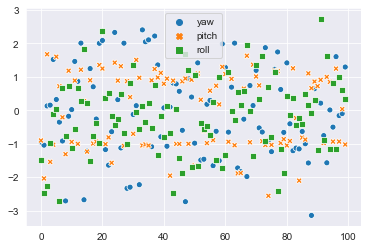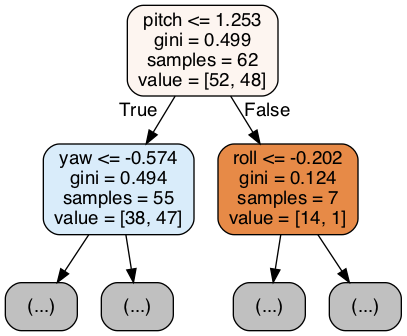### Classification with Random Forests

In :
``````from sklearn.tree import DecisionTreeClassifier
from sklearn.ensemble import RandomForestClassifier
from utils import load_data, draw_tree
import seaborn as sns
sns.set_style("darkgrid", {"figure.facecolor": '#cccccc00'})``````

We begin by importing the data. For this example, we'll use three columns: "yaw", "pitch" and "roll".

In :
``````cols = ['yaw', 'pitch', 'roll']
X, y = load_data(cols)
``````
In :
``sns.scatterplot(data=X);``Training a random forest is as simple as instantiating a class and invoking the `fit` function.

In :
``````c = RandomForestClassifier()
c.fit(X, y);
c.feature_importances_
``````
Out:
``array([0.55562498, 0.2670924 , 0.17728262])``

Visual inspection of the tree lets is possible using graphviz.

In :
``draw_tree(c.estimators_, cols)``
Out:In :
``import jovian``
In [ ]:
``jovian.commit()``
```[jovian] Saving notebook.. ```
In [ ]:
`` ``